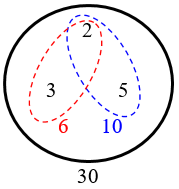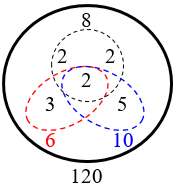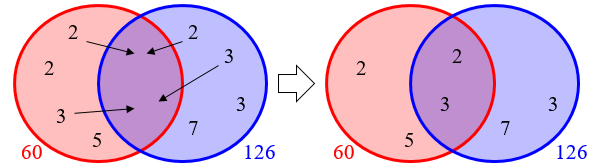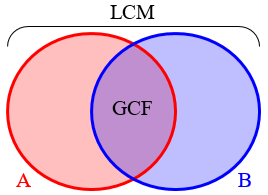# Many Ways to Find the Least Common Multiple

Last time, we looked at three ways to find a GCF (Greatest Common Factor). Here we’ll see the corresponding ways to find an LCM (Least Common Multiple); next time we’ll examine another method, which in its full form finds both at once. (Note that the Least Common Denominator of fractions is just the Least Common Multiple of the denominators, so you will see LCD sometimes used here.) Many of these techniques also illuminate the corresponding ways to find the GCF, and indeed can be used to find both.

## Summary of the three main ways

3 Methods for Finding Least Common Denominator

My fifth graders are having a very difficult time understanding how to get the least common denominator.  Any shortcuts?  Thank you.

Doctor Rob answered, starting with how to handle more than two numbers:

Shortcuts, no.  I use three methods when faced with a problem like this.

First of all, the least common denominator is a multiple of all the denominators. In fact it is the least common multiple (LCM) of all the denominators. One can find the LCM of a set of numbers by doing it two numbers at a time. Find the LCM of the first two numbers. Then find the LCM of that result and the third number. That will be the LCM of the first three numbers. Now find the LCM of this result and the fourth number, and so on. This reduces the problem to dealing with just two numbers at a time.

We’ll see ways to handle more than two numbers at once later, but it is never necessary. That can keep things (relatively) simple.

First method:
Write down the multiples of the two numbers in two lists. Find the numbers which are on both lists, and pick the smallest.

Example: LCM of 12 and 18.

Multiples of 12 are {12, 24, 36, 48, 60, 72, ...}.
Multiples of 18 are {18, 36, 54, 72, ...}.

Numbers common to both lists are {36, 72, ...}.  The smallest is 36, the answer.

This, like the first method for GCF, applies the definition directly, and so is important for one’s first exposure to the concept. But it works best when the LCM will be small.

Our Method 2 is “Prime factors”:

Second method:
Factor the two numbers into products of powers of prime numbers.  Create a new number multiplying together all the primes occurring in either number, raised to the higher of the two exponents.  That is the LCM.

Example:  LCM of 45 and 12.  45 = 3^2*5, 12 = 2^2*3.
New number = 2^2*3^2*5 = 180, the LCM sought.

I’ll be showing four variations of this; this version is the last and most sophisticated. We’ll be seeing why it works.

Our Method 3 is “Use the GCF”:

Third method:
Find the greatest common divisor of the two numbers. Multiply the two numbers together and divide by the greatest common divisor. (You have to know how to find the greatest common divisor to be able to use this method. Do your students know how to do that?)

Example:  LCM of 45 and 12.  Greatest common divisor is 3.  LCM is 45*12/3 = 180.

(Recall that GCF and GCD are names for the same thing.)

This is based on the fact that $$\text{LCM}(A,B)=\frac{AB}{\text{GCF}(A,B)}$$ Below, we’ll see why this works. For very large numbers, you can use the Euclidean algorithm to find the GCF – this method lets us use that very powerful tool to “kill two birds with one algorithm”.

Now for details, which will come mixed together in various answers we’ve given.

## 1: Listing multiples

The next question asks specifically about the prime factor method (2), but the answer starts with the first:

Finding Least Common Multiple (LCM) by Factoring

My math book has the example:

Find the least common multiple of 6, 8, and 9.

6 = 2 x 3   8 = 2 cubed    9 = 3 squared

the least common multiple of 6, 8, and 9 is
2 cubed x 3 squared, or 72.

I understand that 8 x 9 = 72, but what happened to the 6 or 2 x 3? I just don't get how to "figure prime factors and find the product of the greatest power of each prime factor."

Doctor Terrel first shows that merely listing multiples gets difficult even for three small numbers, though it is very important for understanding:

Hi Laura,

Yes, LCM is often a tricky thing to understand, especially going at it via prime factorizations first. Remember what LCM stands for in the beginning...

M = multiple     C = common      L = least (or lowest)

MULTIPLES:
9 ==> 9, 18, 27, 36, 45, 54, 63, 72, 81, 90, 99, ...
8 ==> 8, 16, 24, 32, 40, 48, 56, 64, 72, 80, 88, ...
6 ==> 6, 12, 18, 24, 30, 36, 42, 48, 54, 60, 66, 72, 78, ...

COMMON:
Try to find a number or number(s) that appear in ALL 3 LINES. In the above sample, we only find 72. There are other larger ones, but it takes too long to write them.

LEAST [smallest, first, etc.]
That has to be 72, this time.

This illustrates the method well, and with three numbers at once. But when the LCM is large, you can see how hard it can get; that’s where primes come in.

### 2a: Prime factors (cumulative method)

There are several different ways to use prime factors, and he now gives the one that I think is easiest to understand initially:

Now via PRIME FACTORIZATION:

For 6 it is 2 x 3;
for 8 it is 2 x 2 x 2; and
for 9 it is 3 x 3.

The task now before you is to make a collection or set - as small as possible - of those primes in which you can find all the factors of any given number.

LCM (6, 8, 9) = {2, 3, ...}         [6 is there so far]
= {2, 3, 2, 2, ... }  [two more 2's were needed for 8]
= {2, 3, 2, 2, 3}     [another 3 was needed for the 9]

2 x 3 x 2 x 2 x 3 = 72.

So LCM(6, 8, 9) = 72.

Here we start with the prime factorization of one number, and then try to add in prime factors as needed to make our number a multiple of all the numbers we have.

## More on the cumulative method

For more on this approach, consider this 2003 question:

Finding Least Common Multiple by Using Prime Factors

Can you explain how the prime factorization of a pair of numbers can help you find the least common multiple of those numbers?

Doctor Riz replied:

Suppose you want to find the Least Common Multiple (LCM) of 6 and 10. If we start by factoring them into primes, we get this:

6: 2 * 3
10: 2 * 5

Now the challenge is to find the smallest possible set of prime factors that contains all the factors of each original number.

In other words, we need a 2 and a 3 from the 6.  So far we have 2 * 3.  But we don't need to include another 2 from the 10 since we already have the 2 from the 6.  All we need to add to our set is the 5, so now we have 2 * 3 * 5.  As a check, can you find 2 * 3 in our set of 2 * 3 * 5?  How about 2 * 5?  Yes, both original factor sets are included and there is nothing extra.  So, our LCM is 2 * 3 * 5 or 30.

We can picture the process this way, where we start with the set of factors for 6, then add in the 5 so that we can make 10, but reuse the 2:Let's try another one.  Suppose you are looking for the LCM of 8, 10 and 12.  Start by factoring each one out:

8: 2 * 2 * 2
10: 2 * 5
12: 2 * 2 * 3

Now let's put together the smallest set that contains each of those three sets.  We start with three 2's from the 8.  That gives us:

2 * 2 * 2

For the 10, we certainly don't need another 2 since we already have three of them, but we do need to add in a 5.  Now we have:

2 * 2 * 2 * 5

For the 12, we don't need to do anything with the two 2's because we already have three of them, but we do need to toss in the 3.  Now we have:

2 * 2 * 2 * 5 * 3

Again, can you find each of the three smaller factor sets within that bigger one?  Yes, so we're in business.  The LCM is 2 * 2 * 2 * 5 * 3 or 120.By the way, there is one more thing that's nice about this method.  Suppose you are using it to find the lowest common denominator (LCD) for three fractions with denominators of 8, 10 and 12.  You go through the same steps and wind up with 120.

But now it's time to rename each fraction so it has a denominator of 120.  By looking at the original factor sets versus the common one, you can easily see what each fraction needs to be multiplied by to turn it into a denominator of 120.

For example, since our LCD is 2 * 2 * 2 * 5 * 3 and the fraction with a denominator of 8 already has the 2 * 2 * 2, that one needs to be multiplied by 3 * 5 or 15 to turn it into 120.  The 10 already has the 2 * 5, so it needs to be multiplied by 2 * 2 * 3 or 12 to make 120. Since the 12 already has 2 * 2 * 3, it's missing another 2 and the 5, so it gets multiplied by 2 * 5 or 10 to turn it into 120.  You can see how that can save a little time.

We want to know what times 8 gives 120; the answer is just the product of all the factors outside of 8, which are 3 and 5; and likewise for the others.

We’ll be looking at this use of the LCM in the future.

## An example using two methods

Here’s a 2007 question:

Great Explanation of How to Find the Least Common Multiple

If you set (3) alarm clocks to go off at (3) different times--when will they all ring at the same time?  For example, set clock #1 to ring at 25 min. / clock #2 to ring at 30 min. / clock #3 to ring at 35 min.  How many minutes will it take for them all to ring at the same time?  I was told the answer of 1050 minutes, but I am having trouble figuring out the formula.

This is a common reason to use the LCM; the question ultimately is just about calculating the LCM of 25, 30, and 35.

### 2b: Prime factors (list method)

Doctor Ian answered, first with a second variation of the prime factor method:

Hi Dustin,

The "formula" is really more of a concept.  You want to find the smallest number that is a multiple of 25, 30, and 35, usually called the Least Common Multiple (or LCM) of the numbers.

Multiplying the numbers together gives A common multiple, but not necessarily the LEAST one.  Let's look at the prime factors:

25 =         5 * 5
30 = 2 * 3 * 5
35 =         5     * 7

If we want a number that is a multiple of all these, it would have to have the union of the common factors:

25 =         5 * 5
30 = 2 * 3 * 5
35 =         5     * 7

LCM = 2 * 3 * 5 * 5 * 7 = 1050

So 1050 is, in fact, the LCM.  All three clocks will ring after 1050 minutes, and that is the first time it will happen.

Here we list the prime factors in a row, grouping those that are in all the numbers, and using each of those only once to form the LCM. As we look at other versions, it will become clear why this works; I am calling it the “list” method because we write out lists of primes, and use only one from each column.

### An illustration of cumulative method (2a): shopping for factors

Dustin needed a little more help to see why we do this:

Wow - thank you for the quick and excellent answer.  I am confused on one thing:

I understand the factors

25 = 5*5
30 = 2*3*5
35 = 5*7

But why in the LCM formula, is only 2*3*5*5*7 used and not all the numbers?  What determines the selected numbers?  It seems to me that the formula would include all of the numbers.

Doctor Ian had a nice illustration, likening the cumulative choice of prime factors to shopping:

We want the smallest number that is divisible by 25, 30, and 35.

To be divisible by    We need these prime factors
------------------    ---------------------------
25                    5, 5
30                    2, 3, 5
35                    5, 7

So imagine we have a little shopping basket, and we take it to the 'prime factors store'.  We toss in a couple of 5's, to take care of 25:

basket: 5, 5             <-- We can make 25 from these

Next, we need to 'shop' for 30.  But we already have the 5 we need for 30, right?  All we have to add to our basket are 2 and 3:

basket: 2, 3, 5, 5       <-- We can make 25 from these,
and 30, too.

Finally, we shop for 35.  Again, we already have the 5 we need for this, so we just have to add a 7:

basket: 2, 3, 5, 5, 7    <-- We can make 25 from these,
and 30, too.  And 35.

If we took any one of these prime factors out of the basket, we would lose the ability to make at least one of the numbers.  So this is the smallest possible set of prime factors, and their product is 1050.

Keep in mind that we just want enough to be able to make any one of the numbers 25, 30, and 35, not all of them at once! For that, we’d need the product of all three entire numbers.

We could toss more prime factors in, and while we would get a common multiple, it wouldn't be the LEAST common multiple.  And in fact, that's what happens if we just multiply the numbers--it's like shopping for each number independently, without taking into consideration what we've got from the others:

________________________ These we need.
|  |  |  |        |
25 * 30 * 35: 2, 3, 5, 5, 5, 5, 7
|__|_________ These we don't.

Does that make sense?

It did:

Thank you again, the shopping basket did it all for me.  I understand why we would only use certain prime numbers in the equation.  This site rocks!!!

For a discussion of the last point (how some primes could be “wasted”), see:

Finding the LCM of Several Numbers

## 2c: Prime factors (Venn diagram method)

This unarchived question from 2014 shows a method treating the lists of prime factors as sets in a Venn diagram:

I understand how to find the gcf and the lcm using prime factorization. I typically use a Venn diagram to clearly show which prime factors the numbers have in common and which factors they don't. For me, this method clearly shows why a number is the greatest common factor. It is not as clear to me WHY the lcm is arrived at through multiplying all the factors together.

I answered, having seen this method in a Math for Elementary Teachers class I had taught:

I think the method you are using is like this:

Suppose we want to find the gcf and lcm of the following numbers:

60 = 2*2*3*5
126 = 2*3*3*7

We put the prime factors in a Venn diagram, thinking of them as all distinct (as if each factor of either number were put on a separate tile for each time it occurs, so that we have two tiles with 2, etc.):

_________         _________
60          \     /          126
/                X                \
/                 / \                 \
/                /     \                \
/                /       \                \
|       2        |   2   |       3        |
|                |       |                |
|       5        |   3   |       7        |
\                \       /                /
\                \     /                /
\                 \ /                 /
\                X                /
\ _________ /     \ _________ /

The gcf must be a divisor of each number, so it must be the product of prime factors that are in both circles -- the intersection:

GCF = 2 * 3 = 6

The lcm must be a multiple of each number, so it must be a product of all the prime factors in either circle. This is the union:

LCM = 2 * 5 * 2 * 3 * 3 * 7 = 1260

The is, in order to be a multiple of 60, it must contain all those prime factors (2, 5, 2, 3), and in order to be a multiple of 126, it must contain all those prime factors (2, 3, 3, 7). The smallest set of prime factors that contains both sets is the union.

So this method amounts to arranging the “prime tiles” within each circle to put as much as possible into the intersection (overlap). In doing so, the intersection becomes the GCF (as large as possible), while the union becomes the LCM (as small as possible). We might start with nothing in the intersection, and move in everything that appears in both:Can you see how this explains Method 2b, where we collected duplicated primes and used them only once each?

For a puzzle that uses this method (at a time when I hadn’t yet seen it), see:

Unknown Numbers and a Venn Diagram


## Easiest way?

Here is another question that elicited two methods:

LCD, LCM

What is the easiest way to find the least common denominator and least common multiple of two numbers?

### 2d: Prime factors (exponent method)

Doctor Rob first gave another variation on prime factors (the same one we saw in his answer to the first question); I’ve fixed a couple typos:

If the numbers are small, the easiest way is to factor them into prime powers. Then the greatest common divisor (GCD) has all the primes appearing in either of the factorizations raised to the lesser power between the two, and the least common multiple (LCM) is similar but using the greater power.

Example:  The two numbers are 180 and 54.  180 = 2^2*3^2*5^1, and 54 = 2^1*3^3*5^0.  The GCD is 2^1*3^2*5^0 = 18, because the smaller of the exponents of 2 is 1, the smaller of the exponents of 3 is 2, and the smaller of the exponents of 5 is 0.

The LCM is 2^2*3^3*5^1 = 540, because the larger of the exponents of 2 is 2, the larger of the exponents of 3 is 3, and the larger of the exponents of 5 is 1.

I would typically write my work this way: $$180 = 2^2\times 3^2\times 5^1$$ $$54 = 2^1\times 3^3\times 5^0$$ $$GCF = 2^1\times 3^2\times 5^0 = 18$$ $$LCM = 2^2\times 3^3\times 5^1 = 540$$

### 3: GCF (with Euclidean algorithm)

As with the GCF, very large numbers make factoring difficult, so something easier is needed. We already have an efficient way to find the GCF under these conditions; and it turns out that we can use that to find the LCM. Doctor Rob continued:

If the numbers are large, so that factoring them is hard, the best way to find the GCD is using Euclid's Algorithm.  From the larger one, subtract the biggest multiple of the smaller one you can without getting a negative answer. Replace the larger number with the answer you got. Repeat this until the last number computed is zero, and the GCD is the next-to-last number computed.

Example:  What is the GCD of 347236 and 297228?

347236 -  1*297228 = 50008
297228 -  5* 50008 = 47188
50008 -  1* 47188 =  2820
47188 - 16*  2820 =  2068
2820 -  1*  2068 =   752
2068 -  2*   752 =   564
752 -  1*   564 =   188
564 -  3*   188 =     0

The GCD is 188. Be sure to check your work by dividing to make sure that the GCD really does divide the two numbers:  347236/188 = 1847, and 297228/188 = 1581.

Once you have the GCD, the LCM is the product of the two numbers divided by the GCD. Thus in the example, the LCM of 347236 and 297228 is 347236*297228/188 = 549090936.

For more examples of the algorithm, and why it works, see the last post.

For a proof of the relationship used here, see

Proof Regarding LCM

Relationship Between GCF and LCM

But the Venn diagram method makes this almost obvious: If we multiply all the factors in A and all the factors in B, we have multiplied all the factors in the LCM, but have included the factors in the GCF twice, so $$\text{GCF}(A,B)\times\text{LCM}(A,B)=AB,$$ and therefore $$\text{LCM}(A,B)=\frac{AB}{\text{GCF}(A,B)}$$In summary, we have 6 methods, ultimately, though four are closely related:

1. Listing factors: find the first factor that is in all lists
2. Prime factors